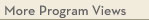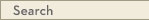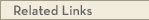# University Catalogs

Twin Cities campusTwin Cities Campus

# Statistics Minor

Statistics, School of
College of Liberal Arts
• Program Type: Undergraduate minor related to major
• Requirements for this program are current for Fall 2019
• Required credits in this minor: 14
Statistics is the science of learning from data; measuring, controlling, and communicating uncertainty. It provides the navigation essential for controlling the course of scientific and societal advances.
Program Delivery
This program is available:
• via classroom (the majority of instruction is face-to-face)
Minor Requirements
Students may earn no more than one undergraduate degree in statistics: a BA or a BS or a minor. Students should take a total of 14 credits, at least 6 of which must be from the Advanced Courses course group.
Introduction to Statistics
Take 0 - 2 course(s) from the following:
· STAT 19xx - Freshman Seminar (3.0 cr)
· Only 1 of the following courses can count towards the minor.
· STAT 3011 - Introduction to Statistical Analysis [MATH] (4.0 cr)
or STAT 3021 - Introduction to Probability and Statistics (3.0 cr)
Intermediate Courses
Take 0 - 4 course(s) from the following:
· STAT 3701 - Introduction to Statistical Computing (4.0 cr)
· STAT 3501 - Internship in Statistical Practice (1.0 cr)
· STAT 3022, 3032, 5302
Only 1 course in the following course pairs can count towards the minor: (STAT 3022 or STAT 3032), (STAT 3032 or STAT 5302). A student may take both STAT 3022 and STAT 5302.
· STAT 3032 - Regression and Correlated Data (4.0 cr)
or Take 0 - 2 course(s) from the following:
· STAT 3022 - Data Analysis (4.0 cr)
· STAT 5302 - Applied Regression Analysis (4.0 cr)
Take 2 or more course(s) totaling 6 or more credit(s) from the following:
· STAT 4051 - Applied Statistics I (4.0 cr)
· STAT 4052 - Introduction to Statistical Learning (4.0 cr)
· STAT 5031 - Statistical Methods for Quality Improvement (4.0 cr)
· STAT 5201 - Sampling Methodology in Finite Populations (3.0 cr)
· STAT 5303 - Designing Experiments (4.0 cr)
· STAT 5401 - Applied Multivariate Methods (3.0 cr)
· STAT 5421 - Analysis of Categorical Data (3.0 cr)
· STAT 5511 - Time Series Analysis (3.0 cr)
· STAT 5601 - Nonparametric Methods (3.0 cr)
· STAT 5931 - Topics in Statistics (3.0 cr)
· STAT 5993 - Tutorial (1.0-6.0 cr)
· Theory of Statistics
At most one of the following course groupings may be included: (STAT 4101 and 4102) or (STAT 5101 and 5102) or (MATH 5651 and STAT 5102).
STAT 4101 - Theory of Statistics I (4.0 cr)
STAT 4102 - Theory of Statistics II (4.0 cr)
or STAT 5101 - Theory of Statistics I (4.0 cr)
STAT 5102 - Introduction to Statistical Learning (4.0 cr)
or MATH 5651 - Basic Theory of Probability and Statistics (4.0 cr)
STAT 5102 - Introduction to Statistical Learning (4.0 cr)View college catalog(s): · College of Liberal Arts View checkpoint chart: · Statistics Minor View PDF Version:Search Programs Search University CatalogsOne Stop for tuition, course registration, financial aid, academic calendars, and moreSTAT 3011 - Introduction to Statistical Analysis (MATH)
 Credits: 4.0 [max 4.0] Course Equivalencies: (Select a set) Typically offered: Every Fall, Spring & Summer
Standard statistical reasoning. Simple statistical methods. Social/physical sciences. Mathematical reasoning behind facts in daily news. Basic computing environment.
STAT 3021 - Introduction to Probability and Statistics
 Credits: 3.0 [max 3.0] Typically offered: Every Fall, Spring & Summer
This is an introductory course in statistics whose primary objectives are to teach students the theory of elementary probability theory and an introduction to the elements of statistical inference, including testing, estimation, and confidence statements. prereq: Math 1272
STAT 3701 - Introduction to Statistical Computing
 Credits: 4.0 [max 3.0] Grading Basis: A-F only Typically offered: Every Fall & Spring
Elementary Monte Carlo, simulation studies, elementary optimization, programming in R, and graphics in R. Prerequisites: (MATH 1272 or 1372 or 1572H), CSCI 1113, and STAT 3032
STAT 3501 - Internship in Statistical Practice
 Credits: 1.0 [max 2.0] Prerequisites: Statistics Major Grading Basis: S-N only Typically offered: Every Fall, Spring & Summer
Internship for statistics undergraduate students, in the university or in the community with supervision provided by statistics faculty and on-site mentors. prereq: Statistics Major
STAT 3032 - Regression and Correlated Data
 Credits: 4.0 [max 4.0] Typically offered: Every Fall & Spring
This is a second course in statistics with a focus on linear regression and correlated data. The intent of this course is to prepare statistics, economics and actuarial science students for statistical modeling needed in their discipline. The course covers the basic concepts of linear algebra and computing in R, simple linear regression, multiple linear regression, statistical inference, model diagnostics, transformations, model selection, model validation, and basics of time series and mixed models. Numerous datasets will be analyzed and interpreted using the open-source statistical software R. prereq: STAT 3011 or STAT 3021
STAT 3022 - Data Analysis
 Credits: 4.0 [max 4.0] Typically offered: Every Fall & Spring
Practical survey of applied statistical inference/computing covering widely used statistical tools. Multiple regression, variance analysis, experiment design, nonparametric methods, model checking/selection, variable transformation, categorical data analysis, logistic regression. prereq: 3011 or 3021 or SOC 3811
STAT 5302 - Applied Regression Analysis
 Credits: 4.0 [max 4.0] Typically offered: Every Fall, Spring & Summer
Simple, multiple, and polynomial regression. Estimation, testing, prediction. Use of graphics in regression. Stepwise and other numerical methods. Weighted least squares, nonlinear models, response surfaces. Experimental research/applications. prereq: 3032 or 3022 or 4102 or 5021 or 5102 or instr consent
STAT 4051 - Applied Statistics I
 Credits: 4.0 [max 4.0] Grading Basis: A-F or Aud Typically offered: Every Fall & Spring
This is the first semester of the Applied Statistics sequence for majors seeking a BA or BS in statistics. The course introduces a wide variety of applied statistical methods, methodology for identifying types of problems and selecting appropriate methods for data analysis, to correctly interpret results, and to provide hands-on experience with real-life data analysis. The course covers basic concepts of single factor analysis of variance (ANOVA) with fixed and random effects, factorial designs, analysis of covariance (ANCOVA), repeated measures analysis with mixed effect models, principal component analysis (PCA) and multidimensional scaling, robust estimation and regression methods, and rank tests. Numerous datasets will be analyzed and interpreted, using the open-source statistical software R and Rstudio. prerequisites: Stat 3032 and Stat 3701 and Stat 4101 or 5101, Math 5651
STAT 4052 - Introduction to Statistical Learning
 Credits: 4.0 [max 4.0] Grading Basis: A-F only Typically offered: Every Fall & Spring
This is the second semester of the core Applied Statistics sequence for majors seeking a BA or BS in statistics. Both Stat 4051 and Stat 4052 are required in the major. The course introduces a wide variety of applied statistical methods, methodology for identifying types of problems and selecting appropriate methods for data analysis, to correctly interpret results, and to provide hands-on experience with real-life data analysis. The course covers basic concepts of classification, both classical methods of linear classification rules as well as modern computer-intensive methods of classification trees, and the estimation of classification errors by splitting data into training and validation data sets; non-linear parametric regression; nonparametric regression including kernel estimates; categorical data analysis; logistic and Poisson regression; and adjustments for missing data. Numerous datasets will be analyzed and interpreted, using the open-source statistical software R and Rstudio. prerequisites: Stat 4051 and Stat 4102 or 5102
STAT 5031 - Statistical Methods for Quality Improvement
 Credits: 4.0 [max 4.0] Typically offered: Periodic Spring
Random variability/sampling. Controlling statistical process. Shewhart/accumulative charting. Analyzing plant data, trend surface, and variance/design of experiments. prereq: [3021 or 3022 or 4102 or 5021 or 5102 or 8102], Math 1272
STAT 5201 - Sampling Methodology in Finite Populations
 Credits: 3.0 [max 3.0] Typically offered: Every Spring
Simple random, systematic, stratified, unequal probability sampling. Ratio, model based estimation. Single stage, multistage, adaptive cluster sampling. Spatial sampling. prereq: 3022 or 4102 or 5021 or 5102 or instr consent
STAT 5303 - Designing Experiments
 Credits: 4.0 [max 4.0] Typically offered: Every Fall, Spring & Summer
Analysis of variance. Multiple comparisons. Variance-stabilizing transformations. Contrasts. Construction/analysis of complete/incomplete block designs. Fractional factorial designs. Confounding split plots. Response surface design. prereq: 3022 or 4102 or 5021 or 5102 or instr consent
STAT 5401 - Applied Multivariate Methods
 Credits: 3.0 [max 3.0] Typically offered: Periodic Fall
Bivariate and multivariate distributions. Multivariate normal distributions. Analysis of multivariate linear models. Repeated measures, growth curve and profile analysis. Canonical correlation analysis. Principal components and factor analysis. Discrimination, classification, and clustering.
STAT 5421 - Analysis of Categorical Data
 Credits: 3.0 [max 3.0] Typically offered: Every Fall & Spring
Varieties of categorical data, cross-classifications, contingency tables. Tests for independence. Combining 2x2 tables. Multidimensional tables/loglinear models. Maximum-likelihood estimation. Tests for goodness of fit. Logistic regression. Generalized linear/multinomial-response models.
STAT 5511 - Time Series Analysis
 Credits: 3.0 [max 3.0] Typically offered: Every Fall
Characteristics of time series. Stationarity. Second-order descriptions, time-domain representation, ARIMA/GARCH models. Frequency domain representation. Univariate/multivariate time series analysis. Periodograms, non parametric spectral estimation. State-space models. prereq: Theoretical understanding, STAT 4102 or STAT 5102
STAT 5601 - Nonparametric Methods
 Credits: 3.0 [max 3.0] Typically offered: Every Fall & Spring
Order statistics. Classical rank-based procedures (e.g., Wilcoxon, Kruskal-Wallis). Goodness of fit. Topics may include smoothing, bootstrap, and generalized linear models. prereq: Stat classes 3032 or 3022 or 4102 or 5021 or 5102 or instr consent
STAT 5931 - Topics in Statistics
 Credits: 3.0 [max 3.0] Typically offered: Periodic Fall
Topics vary according to student needs and available staff.
STAT 5993 - Tutorial
 Credits: 1.0 -6.0 [max 12.0] Typically offered: Every Fall, Spring & Summer
Directed study in areas not covered by regular offerings. prereq: instr consent
STAT 4101 - Theory of Statistics I
 Credits: 4.0 [max 4.0] Typically offered: Every Fall
Random variables/distributions. Generating functions. Standard distribution families. Data summaries. Sampling distributions. Likelihood/sufficiency. prereq: Math 1272 or Math 1372 or Math 1572H
STAT 4102 - Theory of Statistics II
 Credits: 4.0 [max 4.0] Course Equivalencies: 00260 Typically offered: Every Spring
Estimation. Significance tests. Distribution free methods. Power. Application to regression and to analysis of variance/count data. prereq: STAT 4101
STAT 5101 - Theory of Statistics I
 Credits: 4.0 [max 4.0] Typically offered: Every Fall
Logical development of probability, basic issues in statistics. Probability spaces. Random variables, their distributions and expected values. Law of large numbers, central limit theorem, generating functions, multivariate normal distribution. prereq: [Math 2263 or Math 2374 or Math 2573H], [CSCI 2033 or Math 2373 or Math 2243]
STAT 5102 - Introduction to Statistical Learning
 Credits: 4.0 [max 4.0] Course Equivalencies: 00260 Typically offered: Every Spring
Sampling, sufficiency, estimation, test of hypotheses, size/power. Categorical data. Contingency tables. Linear models. Decision theory. prereq: [5101 or Math 5651 or instr consent]
MATH 5651 - Basic Theory of Probability and Statistics
 Credits: 4.0 [max 4.0] Course Equivalencies: 00259 - MATH 4653/Math 5651/Stat 5101 Typically offered: Every Fall & Spring
Logical development of probability, basic issues in statistics. Probability spaces, random variables, their distributions/expected values. Law of large numbers, central limit theorem, generating functions, sampling, sufficiency, estimation. prereq: [2263 or 2374 or 2573], [2243 or 2373]; [2283 or 2574 or 3283] recommended.
STAT 5102 - Introduction to Statistical Learning
 Credits: 4.0 [max 4.0] Course Equivalencies: 00260 Typically offered: Every Spring
Sampling, sufficiency, estimation, test of hypotheses, size/power. Categorical data. Contingency tables. Linear models. Decision theory. prereq: [5101 or Math 5651 or instr consent]